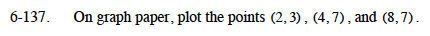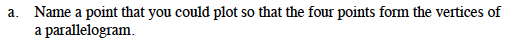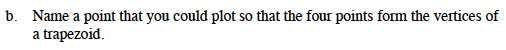### Home > MC1 > Chapter 6 > Lesson 6.3.2 > Problem6-137

6-137.
1. . On graph paper, plot the points (2,3), (4,7), and (8,7). 6-137 HW eTool (Desmos). Homework Help ✎

1. Name a point that you could plot so that the four points form the vertices of a parallelogram.

2. Name a point that you could plot so that the four points form the vertices of a trapezoid.Remember, coordinate points are written in the form (x, y).Remember, a parallelogram is a quadrilateral with both pairs of opposite sides parallel and of equal length.A trapezoid is a quadrilateral with at least one pair of parallel sides.

Use the eTool below to solve the problem.
Click the link at right for the full version of the eTool: MC1 6-137 HW eTool